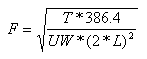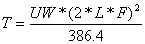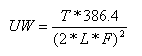# Pitch (Frequency of Vibration), String Tension, String Length, and String Weight

String tension is an important issue for designers of stringed musical instruments, as all the static forces bearing on the structure of an instrument are due to string tension. The relationship of string tension, pitch, and scale length is of interest to all designers of instruments. The tension of a musical instrument string is a function of its mass (weight) per unit of length, the vibrating length of the string, and the pitch of the note produced when the string vibrates. What may not at first appear obvious is that this relationship holds not only for strings of homogeneous construction (i.e. plain strings) but for wound strings as well. This page contains various formulas involving string tension, frequency, string length, and string weight, using U.S. Customary System (USCS) units of measurement. You'll also find javascript calculators for those equations, and some links to other string tension related tools and information. There is also some info for non-technical folks that may help in understanding the relationships between string tension and other quantities.

Those interested in this subject may also want to take a look at Human Perception of String Tension and Compliance in Stringed Musical Instruments, which discusses how players actually perceive string tension and compliance.

Last updated: June 17, 2023

## Frequency of Vibration Formula and Calculator

The formula for determining frequency of vibration using U.S. customary units of measurement is:

### Calculating Frequency of Vibrationwhere:

 F = frequency of vibration, in Hz; T = Tension, in pounds; UW = unit weight of the string, in pounds per linear inch; L = vibrating length of the string (for an open string, this would be the scale length) in inches; Javascript must be enabled in your browser to use the calculator.

Information on unit weights of various types and sizes of strings is available from D'Addario Strings in a document entitled Everything You Ever Wanted to Know About String Tension (.pdf). Click here for a table of notes and their equivalent frequencies.

For the purpose of instrument design it is not often necessary to calculate frequency of vibration from the string properties. So this calculator is that useful in practice. Still, this equation is the master equation from which all the others included here will be derived.

A few things should be apparent from the formula:

1. Everything else being the same, pitch increases as tension increases;

2. Everything else being the same, tension increases as scale length increases;

3. Everything else being the same, tension increases as unit weight increases, thus a heavier gauge string will be under greater tension as a lighter one of the same length tuned to the same note;Given these, it should be obvious why higher pitched instruments tend to have shorter strings and lower pitched instruments longer strings and why fretting a string (i.e. shortening the string) raises the pitch of that string when it vibrates. It also should be obvious why the lower pitched strings of an instrument are wound - this increases the unit weight and thus lowers the pitch. Although it is certainly possible to increase the unit weight of a string simply by increasing its diameter, doing so also increases the string's side bending stiffness. Overly (side bending) stiff strings behave more like bars than strings when they vibrate. Consider what folks in the early 17th century had to do to build an instrument that covered a wide pitch range with a very limited range of available strings and no overspun (wound) strings. The instrument in the picture is called an orpharion. It is related to the bandora and the lute, and is tuned the same as the latter. The splayed frets (also sometimes called fanned frets) make the scale length on the bass side longer than the scale length on the treble side.

## String Tension Formula and Calculator

The formula for determining string tension in U.S. customary units of measurement is:

### Calculating String Tensionwhere:

 T = Tension, in pounds; UW = unit weight of the string, in pounds per linear inch; L = vibrating length of the string (for an open string, this would be the scale length) in inches; F = frequency of the note to which you will tune the string, in Hz; Javascript must be enabled in your browser to use the calculator.
Everything You Ever Wanted to Know About String Tension (.pdf)

This paper is from D'Addario Strings, which surprisingly is the only string manufacturer to provide tension data for all their strings. This paper contains unit weight data for some of their strings, which can be used to approximate unit weights of similarly constructed strings from other manufacturers as well.

The kind folks at D’Addario are both knowledgeable and generous enough to provide us with this data. I thank them by buying their products.

## Unit Weight Formula and Calculator

If you know the scale length, frequency and string tension you can solve for unit weight of the string. This is useful for example in the case where you want to figure out what string to use for, say, the low B string of a seven string guitar. The scale length is the same for the rest of the strings, and you'd want the tension to be more or less the same as the tension of those other strings, too. The frequency is that of the note the open string should sound, in this case low B at 61.735 Hz. Once you determine the unit weight you can look up a string of suitable construction and that unit weight from the D'Addario Everything You Ever Wanted to Know About String Tension (.pdf) document.

The formula for determining unit weight of a string in U.S. customary units of measurement is:

### Calculating Unit Weight of a Stringwhere:

 UW = unit weight of the string, in pounds per linear inch; T = Tension, in pounds; L = vibrating length of the string (for an open string, this would be the scale length) in inches; F = frequency of the note to which you will tune the string, in Hz; Javascript must be enabled in your browser to use the calculator.

## Maximum Tensile Load of Steel Strings Calculator

When designing an instrument it is certainly possible to specify a scale length and a pitch for a string which would require the string to be tensioned past its breaking point. This generally only happens if the instrument is intended to have a relatively long, high pitched string. It is something to consider when designing an instrument from scratch.

The tensile strength (ultimate tensile strength or UTS) of the wire used for plain unwound steel strings and for the cores of wound strings ranges from approximately 350,000 lb/sq.in.to 450,000 lb/sq.in. Hard numbers are not generally available, as string manufacturers tend to consider this information to be proprietary. When designing a new instrument it is best to be conservative and use the low end of this range. From this and the diameter of the string (or for a wound string, the diameter of its core) you can calculate the breaking strength of the string.

The formula for determining the approximate minimum breaking strength of a steel string in U.S. customary units of measurement is:

### Calculating Maximum Tensile Load of a Steel String

S = UTS * π * (D / 2)2

where:

 S = breaking load of the string, in pounds; D = string (core) diameter, in inches; UTS = ultimate tensile strength of the string (core) material, in PSI; 350,000 (min.) 400,000 (mean) 450,000 (max.) Javascript must be enabled in your browser to use the calculator.

Note that the calculator above uses the lower limit of the range by default. As a general rule of thumb, instrument strings can be tensioned to a maximum of approximately 80% of their maximum tensile load. It is rare for strings of fretted instruments to be that close to their maximum tensile load, however.